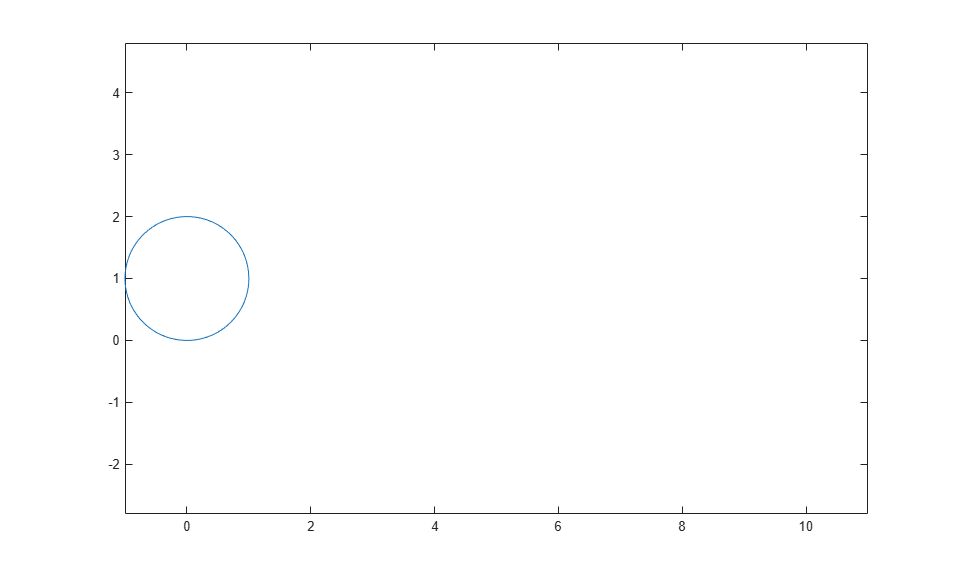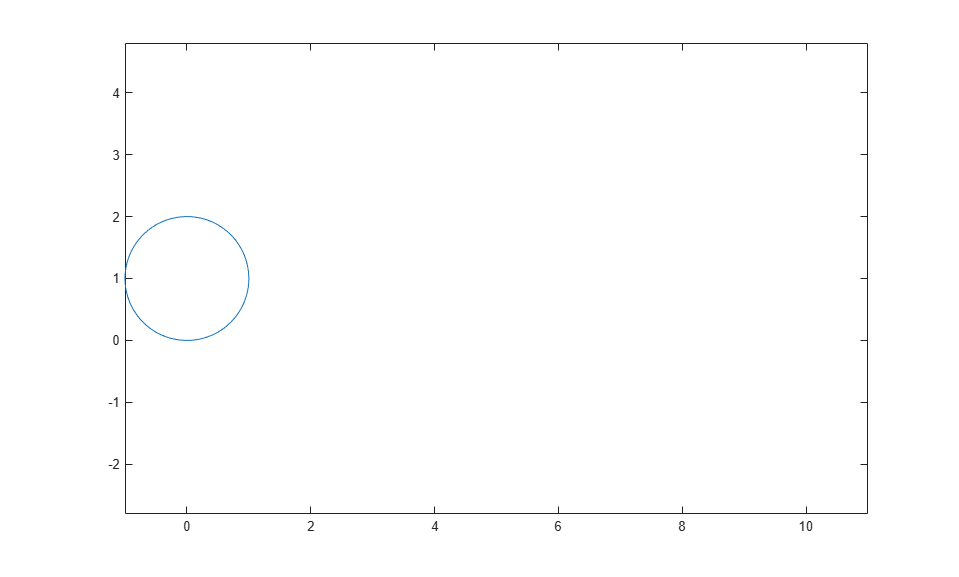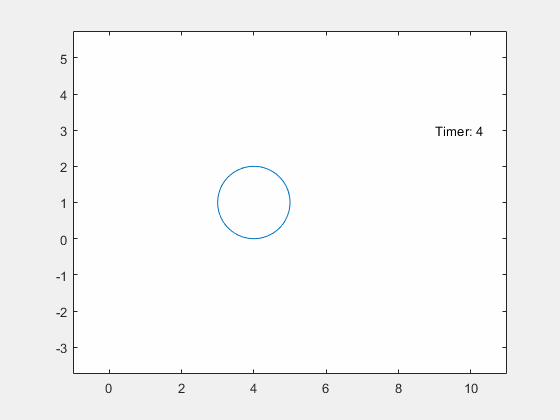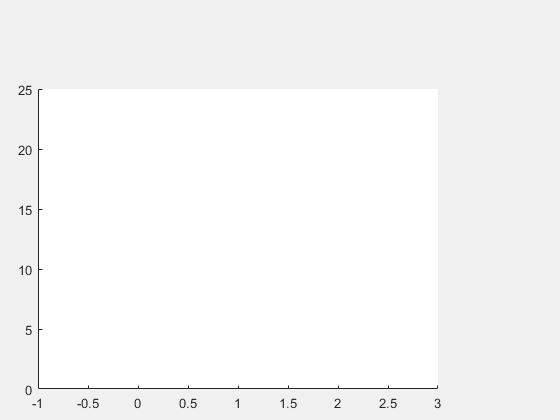playAnimation

Play animation objects in a MATLAB figure window

Description

example

playAnimation plays animation objects in a MATLAB® figure window. The animation objects must be created using the fanimator function.

By default, the variable t = sym('t') is the time parameter of the animation objects. playAnimation plays the animation with 10 frames per unit interval of t within the range of t from 0 to 10.

example

playAnimation(fig) plays animation objects in the figure fig.

example

playAnimation(___,Name,Value) plays the animation objects with the specified Name,Value pair arguments. Use this option with any of the input argument combinations in the previous syntaxes.

Examples

collapse all

First, create an animation object of a moving circle using fanimator.

Create two symbolic variables, t and x. The variable t defines the time parameter of the animation. Use t to set the center of the circle at (t,1) and x to parameterize the perimeter of the circle within the range [-pi pi]. Set the x-axis and y-axis to be equal length.

syms t x
fanimator(@fplot,cos(x)+t,sin(x)+1,[-pi pi])
axis equalNext, enter the command playAnimation to play the animation.By default, playAnimation plays an animation with 10 generated frames per unit time within the range of t from 0 to 10.

Create two symbolic variables, t and x. The variable t defines the time parameter of the animation.

syms t x

Create a circle animation object using fanimator. Use t to set the center of the circle at (t,1) and x to parameterize the perimeter of the circle within the range [-pi pi]. Set the x-axis and y-axis to be equal length.

fanimator(@fplot,cos(x)+t,sin(x)+1,[-pi pi])
axis equalAdd a piece of text to count the elapsed time by using the text function. Use num2str to convert the time parameter to a string.

hold on
fanimator(@(t) text(9,3,"Timer: "+num2str(t,2)))
hold offBy default, playAnimation plays the animation with 10 generated frames per unit time within the range of t from 0 to 10. Change the range of the time parameter to [4 8] using the 'AnimationRange' property. Change the frame rate per unit time to 4 using the 'FrameRate' property. Play the animation in the current figure by entering the following command.

playAnimation(gcf,'AnimationRange',[4 8],'FrameRate',4)Create a UI figure. Specify the UI axes of the figure.

fig = uifigure;
ax = uiaxes(fig);Add an animation object to the UI axes using fanimator. Create two symbolic variables, x and t. Plot a curve that grows exponentially as a function of time t within the interval [0 3].

syms x t;
fanimator(ax,@fplot,exp(x),[0 t],'r','AnimationRange',[0 3])Play the animation in the UI figure fig by entering the command playAnimation(fig). Alternatively, you can also use the command playAnimation(ax.Parent).Input Arguments

collapse all

Name-Value Arguments

Specify optional comma-separated pairs of Name,Value arguments. Name is the argument name and Value is the corresponding value. Name must appear inside quotes. You can specify several name and value pair arguments in any order as Name1,Value1,...,NameN,ValueN.

Example: 'Backwards',true,'FrameRate',25

Range of the animation time parameter, specified as a two-element row vector. The two elements must be real values that are increasing.

Example: [-2 4.5]

Frame rate, specified as a positive value. The frame rate defines the number of frames per unit time when playing the animation objects.

Example: 30

Backward option, specified as a logical value (boolean). If you specify the option true, then the function plays the animation backwards.

Example: true

Speed factor, specified as a real nonzero value. The speed factor sets the ratio of one unit interval of the animation time parameter to one second of clock time.

• If you specify a negative value for 'SpeedFactor' and keep the default value 0 (false) for 'Backwards' option, then the function plays the animation backwards with the specified speed factor. For example, playAnimation('SpeedFactor',-1) launches the same animation as playAnimation('Backwards',true).

• If you specify a zero value for 'SpeedFactor', then playAnimation('SpeedFactor',0) launches a still frame indefinitely and does not play any animation.

Example: 2

Tips

• When you create a graph by using a plotting function, such as fplot, MATLAB creates a series of graphics objects. You can then animate a specific property of the graphics objects by using the fanimator and the playAnimation functions. Note that some functions, such as title and xlabel, create text objects that cannot be animated. Instead, use the text function to create text objects that can be animated.